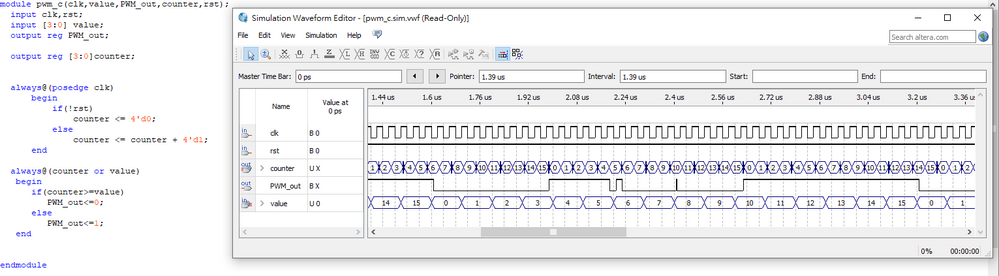FPGA, SoC, And CPLD Boards And Kits
FPGA Evaluation and Development Kits
5408 Discussions

## PWM control problemBeginner
610 Views

Hello guys.

I'm designing a circuit of sort,and the part of PWM is having issue,don't know why...

So I'll briefly describe my PWM specs;

a 4-bit Input named Value,

Input clock 50MHz,

a single bit output PWM_out,

Duty cycle is equal to 1/16 of input clock and

it changes when Value change.

like, Value = 0000, Duty Cycle=0%

Value = 0001,Duty Cycle=6.25%

Value = 0010,Duty Cycle=12.5%

Value = 0010,Duty Cycle=18.75%

.... etc

value changed,duty cycle increased by 6.25%

Here is my code and output doesn't seem correct;

Anyone offers any thoughts and suggests or even help me fix my cod,I would be very grateful.

```module pwm_c(clk,value,PWM_out,counter); input clk; input [3:0] value; output PWM_out; reg PWM_out; output reg [3:0]counter; parameter rst=1; always@(posedge clk) begin if(!rst) counter <= 4'd0; else counter <= counter + 4'd1; end always@(counter or value) begin if(value == 4'd0) PWM_out = 1'b0; else if(value == 4'd1) PWM_out = (counter >=4'd1) ? 1'b0:1'b1; else if(value == 4'd2) PWM_out = (counter >=4'd2) ? 1'b0:1'b1; else if(value == 4'd3) PWM_out = (counter >= 4'd3) ? 1'b0:1'b1; else if(value == 4'd4) PWM_out = (counter >= 4'd4) ? 1'b0:1'b1; else if(value == 4'd5) PWM_out = (counter >= 4'd5) ? 1'b0:1'b1; else if(value == 4'd6) PWM_out = (counter >= 4'd6) ? 1'b0:1'b1; else if(value == 4'd7) PWM_out = (counter >= 4'd7) ? 1'b0:1'b1; else if(value == 4'd8) PWM_out = (counter >= 4'd8) ? 1'b0:1'b1; else if(value == 4'd9) PWM_out = (counter >= 4'd9) ? 1'b0:1'b1; else if(value == 4'd10) PWM_out = (counter >= 4'd10) ? 1'b0:1'b1; else if(value == 4'd11) PWM_out = (counter >= 4'd11) ? 1'b0:1'b1; else if(value == 4'd12) PWM_out = (counter >= 4'd12) ? 1'b0:1'b1; else if(value == 4'd13) PWM_out = (counter >= 4'd13) ? 1'b0:1'b1; else if(value == 4'd14) PWM_out = (counter >= 4'd14) ? 1'b0:1'b1; else if(value == 4'd15) PWM_out = (counter >= 4'd15) ? 1'b0:1'b1; else PWM_out = 1'b0; end endmodule ```

My initial wave simulation of this code;1 SolutionNew Contributor III
244 Views

Where is your test code? That is where control of 'value' comes from. Or did Quartus create that for you? Is that why you can't find where to change it?

I recommend that you use ModelSim - a much more widely use simulation tool. Look at this guide:

The example is similar to the code you're using. Get the test bench right and I think your code will do what you want.

Cheers,

Alex

4 RepliesNew Contributor III
244 Views

It looks approximately right to me. Try changing your 'value' less frequently - every 6 clock cycles (or so) is far too quick. You'd usually expect to set a desired PWM value for multiple PWM cycles (16 clocks per cycle in your case) before changing it.

There are certainly some more elegant ways to code this. However, as I've said your code should do something approximately right. So, modify as above and confirm whether it behaves as you'd expect.

Cheers,

AlexBeginner
244 Views

Where I stuck is exactly don't know how to make `value` to slower,I can't figure out how to make a `input` to change slower to 16 clocks...

Can you give me some ideas?any hint?I've already tried lots ways,but it all end up the same,the PWM has its output but due to `value` changing too fast...it just don't make it right.What I'm trying to achieve...New Contributor III
245 Views

Where is your test code? That is where control of 'value' comes from. Or did Quartus create that for you? Is that why you can't find where to change it?

I recommend that you use ModelSim - a much more widely use simulation tool. Look at this guide:

The example is similar to the code you're using. Get the test bench right and I think your code will do what you want.

Cheers,

AlexBeginner
244 Views

I forgot to update my situation,In the end...silly me...I set the wrong simulation time,as I set the simulation time between 320ns,the result was perfect.

Thanks for the recommendation information,I'll definitely check it out.